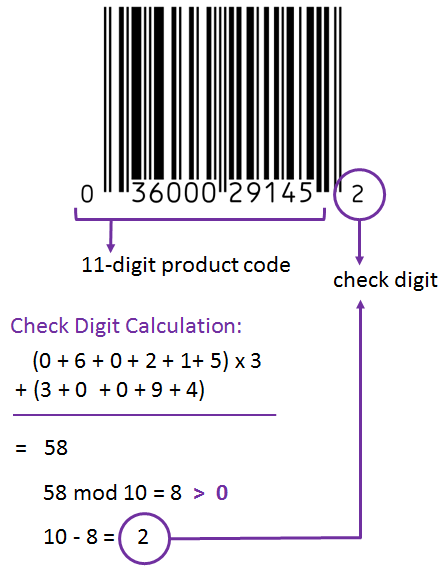Jeremy Davis
Sitecore, C# and web developmentJeremy Davis
Sitecore, C# and web development

# Repeatable delays from data

Published 02 August 2021

I had an interesting discussion recently, about how some code could calculate a "random" but deterministic numeric value based on some data. This made me think of the classic old concept of "checksums" as a possible solution. Here's what I was thinking about:

## The problem

The discussion started because of the need to make some scheduled code run in Kubernetes pods, with multiple instances of the same image running at the same time. But we didn't want the scheduled code to end up starting at exactly the same time in each pod. Because the number of replicas running could vary, there was a desire to avoid setting this delay via explicit config, so we were looking for ways of automating this.

## One possible solution

Each of these pods has a name which comes through to the code as the machine name in environment variables. So if we can compute a delay value based on the name, then we could probably get a reasonable first pass at this. Names of pods are usually a mix of text and numbers, plus some punctuation. So we'll need a bit of maths...

A common code pattern where users need to enter identity data, is to add a digit to the end which represents a checksum. The last digit of a card or account number is a common place to find this – the last digit is computed from the preceding ones to give a reasonable test that all the numbers have been entered correctly. There are a collection of common algorithms for doing this – but I was considering stealing the one from "UPC" barcodes. There, you start with a multi-digit number and:• Add together the values of the odd digits
• Multiply that value by three
• Add together the values of the even digits
• Add together the odd and even sums
• Take the remainder of dividing that sum by 10
• If that's not zero subtract it from 10

So you end up with a number that's based on the source, and that will change if the source changes.

## How can that work here?

It could start from the string that is the machine name that the code sees inside the pod:

```var name = System.Net.Dns.GetHostName();
```

And that can be used to work out a value for the delay:

```var delay = CalculateDelay(name);
```

In simple code, calculating that function looks like:

```int CalculateDelay(string name)
{
var integers = name.ToLower().Select(c => (int)c);

var odds = integers.Where((c, i) => i % 2 != 0).Sum() * 3;
var evens = integers.Where((c, i) => i % 2 == 0).Sum();

var sum = (odds + evens) % 10;

if(sum > 0)
{
sum = 10 - sum;
}

return sum;
}
```

It's probably not ideal to be adding numbers as large as this using the UPC algorithm, but it seems to work. So for any machine name we'll end up with a delay value from zero to ten. Taking a set of possible pod names where the code might run, the outputs look like:

```cm-ae411c6fd6-yttss -> 8s    cm-0625876ce1-ptpdo -> 4s
cm-33b99ec5f0-hklfy -> 3s    cm-d10b43095b-ofxnf -> 9s
cm-b61a9a47b4-rhnrz -> 7s    cm-182f39205e-qbgat -> 4s
cm-99f646c98d-hbfye -> 0s    cm-579c2e34d5-tndcc -> 9s
cm-5033a8d1bb-dhrnr -> 5s    cm-504e5ccdae-oygnu -> 9s
cm-8ce2fdf292-ynwid -> 6s    cm-245fd1273e-zwdia -> 2s
cm-6e2131b125-wcigd -> 4s    cm-135354ddf2-qlhjc -> 1s
cm-fc84f3b0f6-fifbq -> 4s    cm-3743d96305-fxtto -> 5s
cm-d1e9220abd-emvke -> 2s    cm-4630ff27d7-qfubm -> 6s
cm-f4653da2ef-dcehw -> 3s    cm-6f34d6f2c9-ritip -> 9s
```

That gives a reasonable spread of values for whatever string we the code starts from, and they're repeatable rather than random.